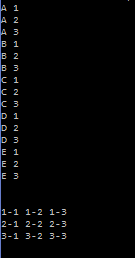# Assignemnt #111, Nesting Loops

## Code

```public class NestingLoops
{
public static void main( String[] args )
{
// this is #1 - I'll call it "CN"
for ( char c='A'; c <= 'E'; c++ )
{
for ( int n=1; n <= 3; n++ )
{
System.out.println( c + " " + n );// The inner loop is faster and the variable is controlled by the outer loop
}// When c is on the inside the letters change before the numbers
}

System.out.println("\n");

// this is #2 - I'll call it "AB"
for ( int a=1; a <= 3; a++ )
{
for ( int b=1; b <= 3; b++ )
{
System.out.print( a + "-" + b + " " );// If you change this print to a println then the output goes on a new line every time
}
System.out.println();// This adds a new line every 3 iterations
}

System.out.println("\n");

}
}

```

### Picture of the output